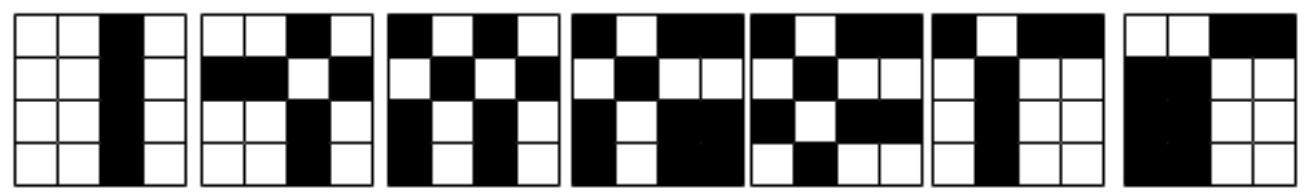시간 제한메모리 제한제출정답맞힌 사람정답 비율
1.5 초 512 MB84413452.308%

## 문제

You are given a matrix of size 2n × 2n, initially painted in white color. The color of a cell can be either black or white. Let’s define the price of a matrix as:

1. If a matrix is painted with only one color, the price will be 1 coin;
2. Otherwise, you should split the matrix into 4 size-equal matrices, and the price of a matrix will be the sum of submatrices prices plus 1 coin.

You are given q queries. Each query gives you the number of row/column x, and you have to change the color of all cells in this row/column (i.e., if a cell is white, it will be black; if a cell is black, it will be white) and find the price of the new matrix.

## 입력

The first line contains two integers n and q (0 ≤ n ≤ 20, 1 ≤ q ≤ 106) where n means that the size of the matrix is 2n × 2n and q means that there are going to be q queries.

Each of the next q lines contains two integers t and x (0 ≤ t ≤ 1, 1 ≤ x ≤ 2n). If t = 0, then the x-th row will be changed; otherwise, the x-th column.

## 출력

For each query, print a matrix price.

## 예제 입력 1

2 7
1 3
0 2
1 1
1 4
0 4
0 3
1 1


## 예제 출력 1

13
17
21
17
21
17
13


## 힌트

In the sample, after each query the matrix will be as follows: